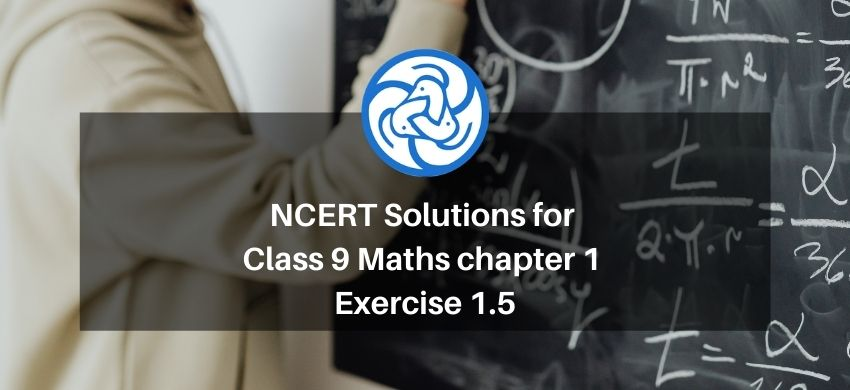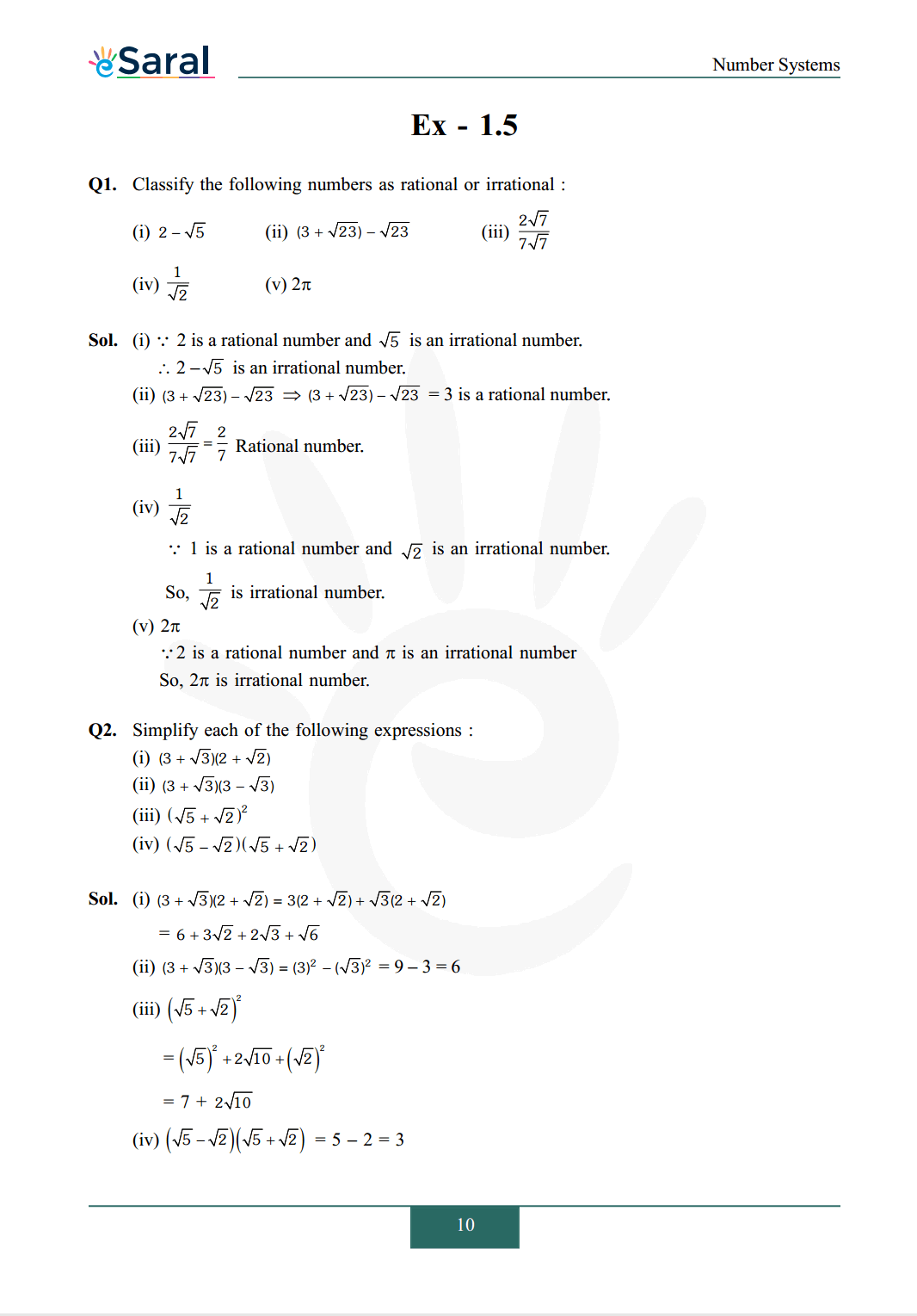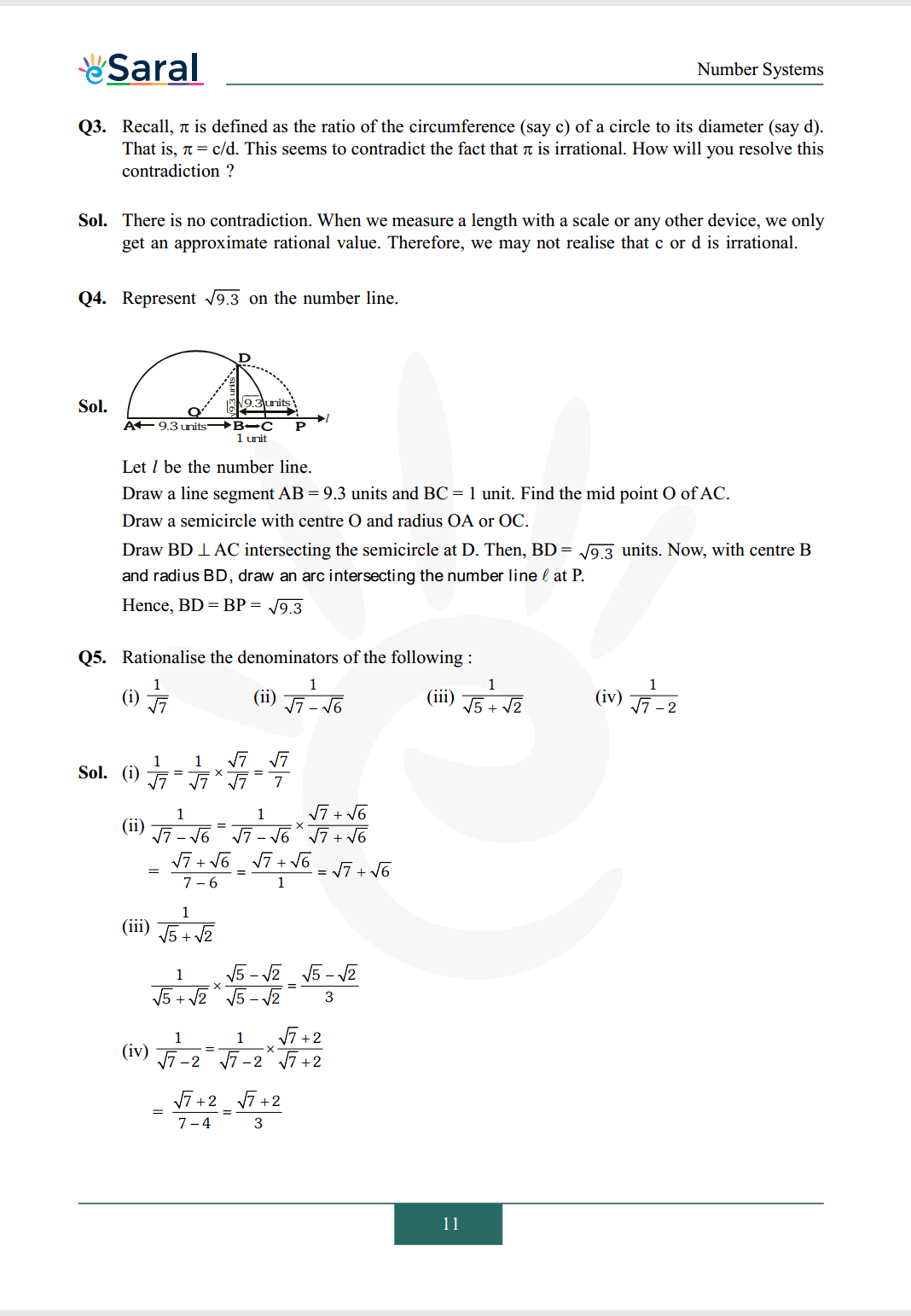# NCERT Solutions for Class 9 Maths chapter 1 Exercise 1.5 - Number System - Free PDF DownloadHey, are you a class 9 Student and Looking for Ways to Download NCERT Solutions for Class 9 Maths chapter 1 Exercise 1.5? If Yes then you are at the right place.

Here we have listed Class 9 maths chapter 1 exercise 1.5 solutions  in PDF that is prepared by Kota’s top IITian’s Faculties by keeping Simplicity in mind.

If you want to score high in your class 9 Maths Exam then it is very important for you to have a good knowledge of all the important topics, so to learn and practice those topics you can use eSaral NCERT Solutions.

So, without wasting more time Let’s start.

### Download The PDF of NCERT Solutions for Class 9 Maths chapter 1 Exercise 1.5 "Number System"#### All Questions of Chapter 1 Exercise 1.5

Once you complete the chapter 1 then you can revise Ex. 1.5 by solving following questions

Q1. Classify the following numbers as rational or irrational:
(i) $2-\sqrt{5}$
(ii) $(3+\sqrt{23})-\sqrt{23}$
(iii) $\frac{2 \sqrt{7}}{7 \sqrt{7}}$
(iv) $\frac{1}{\sqrt{2}}$
(v) $2 \pi$

Q2. Simplify each of the following expressions:
(i) $(3+\sqrt{3})(2+\sqrt{2})$
(ii) $(3+\sqrt{3})(3-\sqrt{3})$
(iii) $(\sqrt{5}+\sqrt{2})^{2}$
(iv) $(\sqrt{5}-\sqrt{2})(\sqrt{5}+\sqrt{2})$

Q3. Recall, $\pi$ is defined as the ratio of the circumference (say $\mathrm{c}$ ) of a circle to its diameter (say d). That is, $\pi=\mathrm{c} / \mathrm{d}$. This seems to contradict the fact that $\pi$ is irrational. How will you resolve this contradiction?

Q4. Represent $\sqrt{9.3}$ on the number line.

Q5. Rationalise the denominators of the following :
(i) $\frac{1}{\sqrt{7}}$
(ii) $\frac{1}{\sqrt{7}-\sqrt{6}}$
(iii) $\frac{1}{\sqrt{5}+\sqrt{2}}$
(iv) $\frac{1}{\sqrt{7}-2}$

Class 9 Maths Revision Quick Videos.